Next: Rocket science Up: Conservation of momentum Previous: Two-component systems

## Multi-component systems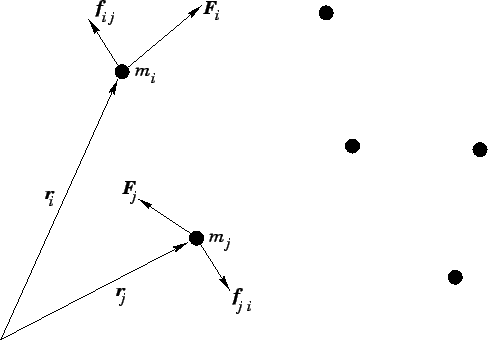Consider a system ofmutually interacting point mass objects which move in 3-dimensions. See Fig. 48. Let the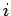th object, whose mass is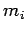, be located at vector displacement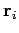. Suppose that this object exerts a forceon the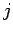th object. By Newton's third law of motion, the force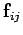exerted by theth object on theth is given by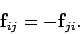(195)

Finally, suppose that theth object is subject to an external force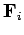.

Newton's second law of motion applied to theth object yields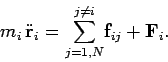(196)

Note that the summation on the right-hand side of the above equation excludes the case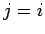, since theth object cannot exert a force on itself. Let us now take the above equation and sum it over all objects. We obtain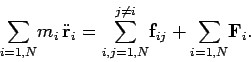(197)

Consider the sum over all internal forces: i.e., the first term on the right-hand side. Each element of this sum--, say--can be paired with another element--, in this case--which is equal and opposite. In other words, the elements of the sum all cancel out in pairs. Thus, the net value of the sum is zero. It follows that the above equation can be written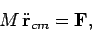(198)

where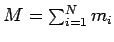is the total mass, and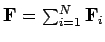is the net external force. The quantity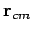is the vector displacement of the centre of mass. As before, the centre of mass is an imaginary point whose coordinates are the mass weighted averages of the coordinates of the objects which constitute the system. Thus,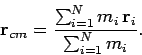(199)

According to Eq. (198), the motion of the centre of mass is equivalent to that which would be obtained if all the mass contained in the system were collected at the centre of mass, and this conglomerate mass were then acted upon by the net external force. As before, the motion of the centre of mass is likely to be far simpler than the motions of the component masses.

Suppose that there is zero net external force acting on the system, so that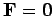. In this case, Eq. (198) implies that the centre of mass moves with uniform velocity in a straight-line. In other words, the velocity of the centre of mass,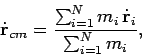(200)

is a constant of the motion. Now, the momentum of theth object takes the form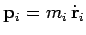. Hence, the total momentum of the system is written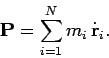(201)

A comparison of Eqs. (200) and (201) suggests thatis also a constant of the motion when zero net external force acts on the system. Finally, Eq. (198) can be rewritten(202)

In other words, the time derivative of the total momentum is equal to the net external force acting on the system.

It is clear, from the above discussion, that most of the important results obtained in the previous section, for the case of a two-component system moving in 1-dimension, also apply to a multi-component system moving in 3-dimensions.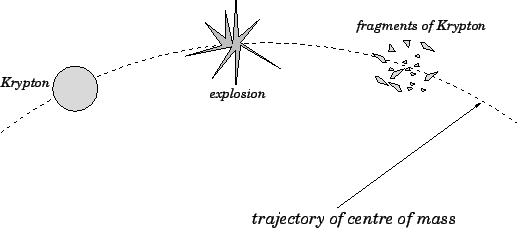As an illustration of the points raised in the above discussion, let us consider the unfortunate history of the planet Krypton. As you probably all know, Krypton--Superman's home planet--eventually exploded. Note, however, that before, during, and after this explosion the net external force acting on Krypton, or the fragments of Krypton--namely, the gravitational attraction to Krypton's sun--remained the same. In other words, the forces responsible for the explosion can be thought of as large, transitory, internal forces. We conclude that the motion of the centre of mass of Krypton, or the fragments of Krypton, was unaffected by the explosion. This follows, from Eq. (198), since the motion of the centre of mass is independent of internal forces. Before the explosion, the planet Krypton presumably executed a standard elliptical orbit around Krypton's sun. We conclude that, after the explosion, the fragments of Krypton (or, to be more exact, the centre of mass of these fragments) continued to execute exactly the same orbit. See Fig. 49.Next: Rocket science Up: Conservation of momentum Previous: Two-component systems
Richard Fitzpatrick 2006-02-02